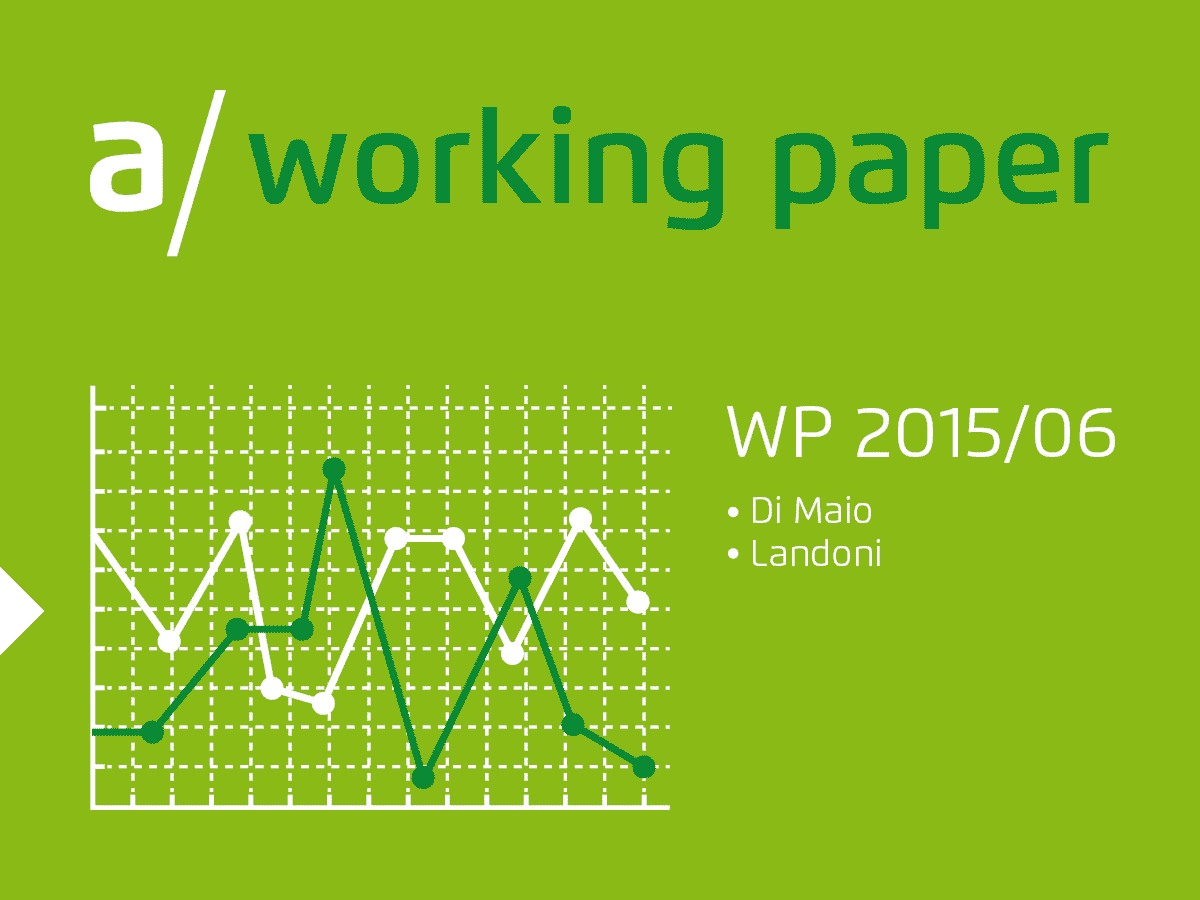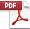# WP 2015/06: Beyond the Gini index. Measuring inequality with the Balance of Inequality index9 Aprile 2015

In the first part of this paper, we present the Balance of Inequality (BOI) index, a new approach to the measurement of inequality in which each individual is given a weight equal to her income, and the barycenter of the income distribution is used to measure its inequality. The BOI index features an intuitive physical interpretation and a simple graphical representation that shows the income distribution and the inequality measure together. The index’s lower and upper bounds are always 0 and 1, which indicates that it is an accurate measure of inequality even when applied to small populations and small samples – cases in which the Gini index is downward biased. We also show that the BOI index is related to the Gini index.In the second part of the paper, we decompose inequality by population subgroups for both the BOI, and Gini indices with a new approach that considers the distribution of the individual members of each subgroup in the population. This decomposition is accomplished by introducing the barycenter of the subgroups for cases of perfect equality and perfect inequality, the asymmetry effect and the irregularity effect. After demonstrating that the BOI index is decomposable by population subgroups, we show that i) the lower and upper bounds of the BOI index and of the Gini index are computable when grouped data or mean incomes are available but the inequalities within subgroups are unknown (without any assumptions regarding the distribution function), ii) the BOI index and the Gini index can be computed with frequency distributions, and iii) the average within-subgroup inequality implied in the Gini indices of the population inequality given with grouped data can be computed. We also show that the Lambert-Aronson (1993) residual i) can be non-null when the subsets do not overlap and ii) is not the effect of the overlapping between subgroups but of the approach used. Finally, we outline the BOI index decomposition by income sources and propose a new formulation of the population principle.

Giorgio Di Maio
Politecnico di Milano
a/simmetrie

Paolo Landoni
Politecnico di Milano

Keywords: Inequality measurement, Gini index, Inequality decomposition by population subgroups, Inequality decomposition by income sources, Balance of Inequality, BOI index; Asymmetry Effect, Irregularity Effect, R concentration ratio.

JEL classification: D31, D63, I32.Condividi